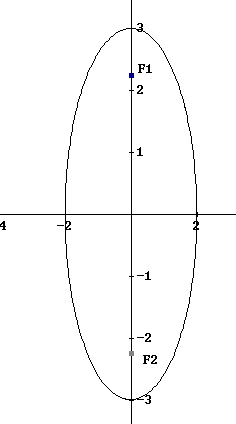# Equation of Ellipse - Problems

This is a tutorial with detailed solutions to problems related to the ellipse equation. An HTML5 Applet to Explore Equations of Ellipses is also included in this website.

## Review

An ellipse with center at the origin (0,0), is the graph ofwith a > b > 0

The length of the major axis is 2a, and the length of the minor axis is 2b. The two foci (foci is the plural of focus) are at (~+mn~ c , 0) or at (0 , ~+mn~ c), where c2 = a2 - b2.

### Problem 1

Given the following equation
9x
2 + 4y2 = 36
a) Find the x and y intercepts of the graph of the equation.
b) Find the coordinates of the foci.
c) Find the length of the major and minor axes.
d) Sketch the graph of the equation.
Solution to Example 1
a)
We first write the given equation in standard form by dividing both sides of the equation by 36 and simplify
9 x
2 / 36 + 4 y2 / 36 = 1
x
2 / 4 + y2 / 9 = 1
x
2 / 22 + y2 / 32 = 1
We now identify the equation obtained with one of the standard equation in the review above and we can say that the given equation is that of an ellipse with a = 3 and b = 2
NOTE: a > b

Set y = 0 in the equation obtained and find the x intercepts.

x2 / 22  = 1
Solve for x.
x2  = 22
x = ~+mn~ 2

Set x = 0 in the equation obtained and find the y intercepts.
y2 / 32 = 1
Solve for y.
y2  = 32
y = ~+mn~ 3
b) We need to find c first.
c2 = a2 - b2
a and b were found in part a)
c2 = 32 - 22
c2 = 5
Solve for c to obtain
c = ~+mn~ (5)1/2
The foci are F1 (0 , √5) and F2 (0 , - √5 )
c) The major axis length is given by 2 a = 6
The minor axis length is given by 2 b = 4
d) Locate the x and y intercepts, find extra points if needed and sketch.Matched Problem: Given the following equation
4x
2 + 9y2 = 36
a) Find the x and y intercepts of the graph of the equation.
b) Find the coordinates of the foci.
c) Find the length of the major and minor axes.
d) Sketch the graph of the equation.

## More Links and References on Ellipses

College Algebra Problems With Answers - sample 8: Equation of Ellipse
Points of Intersection of an Ellipse and a line
HTML5 Applet to Explore Equations of Ellipses
Find the Points of Intersection of a Circle and an Ellipse
Ellipse Area and Perimeter Calculator
Find the Points of Intersection of Two Ellipses